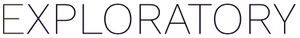# Calculating weights in PCA

#1

Having a look at the PCA analysis in exploratory and have a question about how Exploratory.io calculates the Weights.

Usually I would do this manually by dividing the loading by the square root of the eigenvalue. I just want to make sure that this is the same as what’s being presented in the Weights table.

#2

Hi Carl,

Yes, basically that is what we show there, except that we square them before showing on the Weight table,
so that the values in each row sum up to 1.

#3